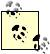# Dealing with Complex Numbers

Problem

You need to perform complex algebra (e.g., when working on electrical engineering problems involving alternating current), and would like to know how Excel supports complex numbers so you can use them in your spreadsheets.

Solution

Excel supports complex numbers in the form a + bi or a + bj (j is often used to indicate a complex number instead of i, as is common in electrical engineering applications). Further, Excel provides 18 built-in functions that can be used to manipulate complex numbers and perform complex algebra.You need to have the Analysis ToolPak loaded in order to use Excel's complex number functions. See the introduction to Chapter 5 for instructions on loading the Analysis ToolPak.

Discussion

In Excel, a complex number is entered into a cell in the form a + bi or a + bj. In reality, Excel treats complex numbers entered this way as text. To enter a complex number, simply type it in as you would text. For example, to enter the complex number 6 + 2j, simply select a cell, type in 6+2j and then press Enter. Or you can use the COMPLEX function to let Excel format the complex number, given the real and imaginary parts you provide. For example, entering the formula =COMPLEX(6,2) in a cell results in the cell displaying the text 6+2i.

Excel provides a suite of special-purpose imaginary number functions to manipulate complex numbers in these forms. There are 18 functions that are designed to work specifically with complex numbers. All but one of these functions start with the prefix IM (for example, IMABS, IMPRODUCT and so on). The one function that does not start with the IM prefix is COMPLEX. These and the other complex number functions are summarized in Table 7-8.

Table 7-8. Complex number functions

Function

Syntax

Description

COMPLEX

=COMPLEX(real part,imaginary part)

Takes the real and imaginary arguments and returns text representing the complex number

IMABS

=IMABS(complex number)

Returns the modulus of the complex number

IMAGINARY

=IMAGINARY(complex number)

Returns the imaginary part of a complex number

IMARGUMENT

=IMARGUMENT(complex number)

Returns the argument Q, in radians, of the complex number, where Q is from the exponential form of complex numbers, reiQ

IMCONJUGATE

=IMCONJUGATE(complex number)

Returns the complex conjugate of a complex number

IMCOS

=IMCOS(complex number)

Returns the cosine of a complex number

IMDIV

=IMDIV(n1, n2)

Returns the quotient of two complex numbers where the first argument is the numerator and the second is the denominator

IMEXP

=IMEXP(complex number)

Returns the exponential of a complex number

IMLN

=IMLN(complex number)

Returns the natural logarithm of a complex number

IMLOG10

=IMLOG10(complex number)

Returns the base 10 logarithm of a complex number

IMLOG2

=IMLOG2(complex number)

Returns the base 2 logarithm of a complex number

IMPOWER

=IMPOWER(complex number, n)

Raises the complex number to the nth power

IMPRODUCT

=IMPRODUCT(n1, n2, n3, ...)

Returns the product of two or more complex numbers

IMREAL

=IMREAL(complex number)

Returns the real part of a complex number

IMSIN

=IMSIN(complex number)

Returns the sine of a complex number

IMSQRT

=IMSQRT(complex number)

Returns the square root of a complex number

IMSUB

=IMSUB(n1, n2)

Subtracts the complex number n2 from n1

IMSUM

=IMSUM(n1, n2, n3, ...)

Returns the sum of two or more complex numbersExcel Scientific and Engineering Cookbook (Cookbooks (OReilly))
ISBN: 0596008791
EAN: 2147483647
Year: N/A
Pages: 206
Authors: David M Bourg